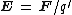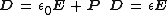# electric field

(redirected from E-field)
Also found in: Dictionary, Thesaurus, Medical.

## electric field

a field of force surrounding a charged particle within which another charged particle experiences a force
Collins Discovery Encyclopedia, 1st edition © HarperCollins Publishers 2005

## Electric field

A condition in space in the vicinity of an electrically charged body such that the forces due to the charge are detectable. An electric field (or electrostatic field) exists in a region if an electric charge at rest in the region experiences a force of electrical origin. Since an electric charge experiences a force if it is in the vicinity of a charged body, there is an electric field surrounding any charged body.

The electric field intensity (or field strength) E at a point in an electric field has a magnitude given by the quotient obtained when the force acting on a test charge q placed at that point is divided by the magnitude of the test charge q. Thus, it is force per unit charge. A test charge q is one whose magnitude is small enough so it does not alter the field in which it is placed. The direction of E at the point is the direction of the force F on a positive test charge placed at the point. Thus, E is a vector point function, since it has a definite magnitude and direction at every point in the field, and its defining equation is Eq. (1).

(1)Electric flux density or electric displacement D in a dielectric (insulating) material is related to E by either of the equivalent equations shown as Eqs. (2),
(2)where P is the polarization of the medium, and ε is the permittivity of the dielectric which is related to ε 0, by the equation ε = k ε 0, k being the relative dielectric constant of the dielectric. In empty space, D = ε 0 E .

In addition to electrostatic fields produced by separations of electric charges, an electric field is also produced by a changing magnetic field. See Electric charge, Electromagnetic induction, Potentials

McGraw-Hill Concise Encyclopedia of Physics. © 2002 by The McGraw-Hill Companies, Inc.
The following article is from The Great Soviet Encyclopedia (1979). It might be outdated or ideologically biased.

## Electric Field

a particular manifestation, along with the magnetic field, of an electromagnetic field that determines the effects upon an electric charge of a force that is independent of the velocity of the charge (see).

The concept of electric field was introduced into science by M. Faraday in the 1830’s. According to Faraday, each stationary charge creates an electric field in the surrounding space. The field of one charge acts upon another charge and vice versa, and an interaction between charges occurs (concept of short-range action). The principal quantitative characteristic of an electric field is its intensity E, defined as the ratio of the force F acting upon the charge to the magnitude of the charge q:E = F/q (seeFIELD INTENSITY, ELECTRIC). The electric field in a medium is characterized not only by its intensity but also by the electric induction vector (seeINDUCTION, ELECTRICAL AND MAGNETIC). The distribution of an electric field in space is visually represented by the intensity lines of force (seeLINES OF FORCE). The lines of force of a potential electric field that is created by electric charges originate on positive charges and terminate on negative charges. The lines of force of a rotational electric field, that is, an electric field created by a changing magnetic field, are closed curves.

The intensity of an electric field satisfies the principle of superposition, according to which for a given point in space, the intensity of the field E, created by several charges, is equal to the sum of the field intensities (E1, E2, E3, . . . .) of the individual charges: E = E1 + E2 + E3 + . . . . The superposition of fields follows from the linearity of Maxwell’s equations (seeMAXWELL’S EQUATIONS).

### REFERENCES

Tamm I. E. Osnovy teorii elektrichestva, 9th ed. Moscow, 1976. Chapters 1 and 6.
Kalashnikov S. G. Elektrichestvo, 4th ed. Moscow, 1977. (Obshchii kura fiziki.) Chapters 2 and 13.

G. IA. MIAKISHEV

## electric field

[i¦lek·trik ′fēld]
(electricity)
One of the fundamental fields in nature, causing a charged body to be attracted to or repelled by other charged bodies; associated with an electromagnetic wave or a changing magnetic field.
Specifically, the electric force per unit test charge.
McGraw-Hill Dictionary of Scientific & Technical Terms, 6E, Copyright © 2003 by The McGraw-Hill Companies, Inc.
References in periodicals archive ?
Most methods shown in Figure 1 employ electric fields normal to the material sample ("out-of-plane"), while the Split-Cylinder Resonator method (IPC-TM-650, 2.5.5.13) used by at least seven laminate manufacturers for their Dk/Df dielectric tables use E-fields tangential to the x-y plane of the laminate ("in plane"), as shown in FIGURE 3.
The broadside E-field magnitude caused by the feedpoint Qc pulse is plotted in Figure 8 where an
(i) Experimental studies (in situ measurements) into the LIC (at ankle) in volunteers staying close to EMR source and mapping the E-field spatial distribution near the source to create and validate the numerical model of this EMR source and the EMR exposure scenario to be used in in silico measurements
In FDTD, a space grid is introduced in which E-field components and H-filed components at different directions are discretized, so each E-filed component is encircled by four H-field components, and also each H-filed component is encircled by four E-field components.
Due to the fact that the fundamental mode in the Coaxial Transmission Line is a TEM mode, E-field components extend from the central conductor to the external ground plane conductor, perpendicular to the conductor surfaces, whereas the H-field lines close around the central conductor strip in a concentric manner.
Dipole antenna Dimension(mm) Dipole length 149mm source 1mm Dipole radius 3.6mm Table 3: Comparison between E-field strength between two model (human head and PIFA and dipole antenna).
A mutual coupling compensation model based on near E-field monitors has been implemented.
Figure 5 shows that the E-field simulated using Shim and Hubing method is reasonably close to the E-field simulated using the original configuration only at low frequencies.
This antenna has been measured at 9.41 GHz and the E-field has been evaluated at 350 mm from the horn.
It follows that the higher contrast images are being governed by the E-field direction.
In this study, we show how by tuning the diameter and the gap to control the transmission and E-field enhancement properties of periodic hexagonal lattice arrays of silver nanodisks through the interactions of nanodisks.
The MGC3130 is presented as the world's first electrical-field (E-field)-based, configurable 3D gesture controller, offering low-power, precise, fast and robust hand position tracking with free-space gesture recognition.

Site: Follow: Share:
Open / Close